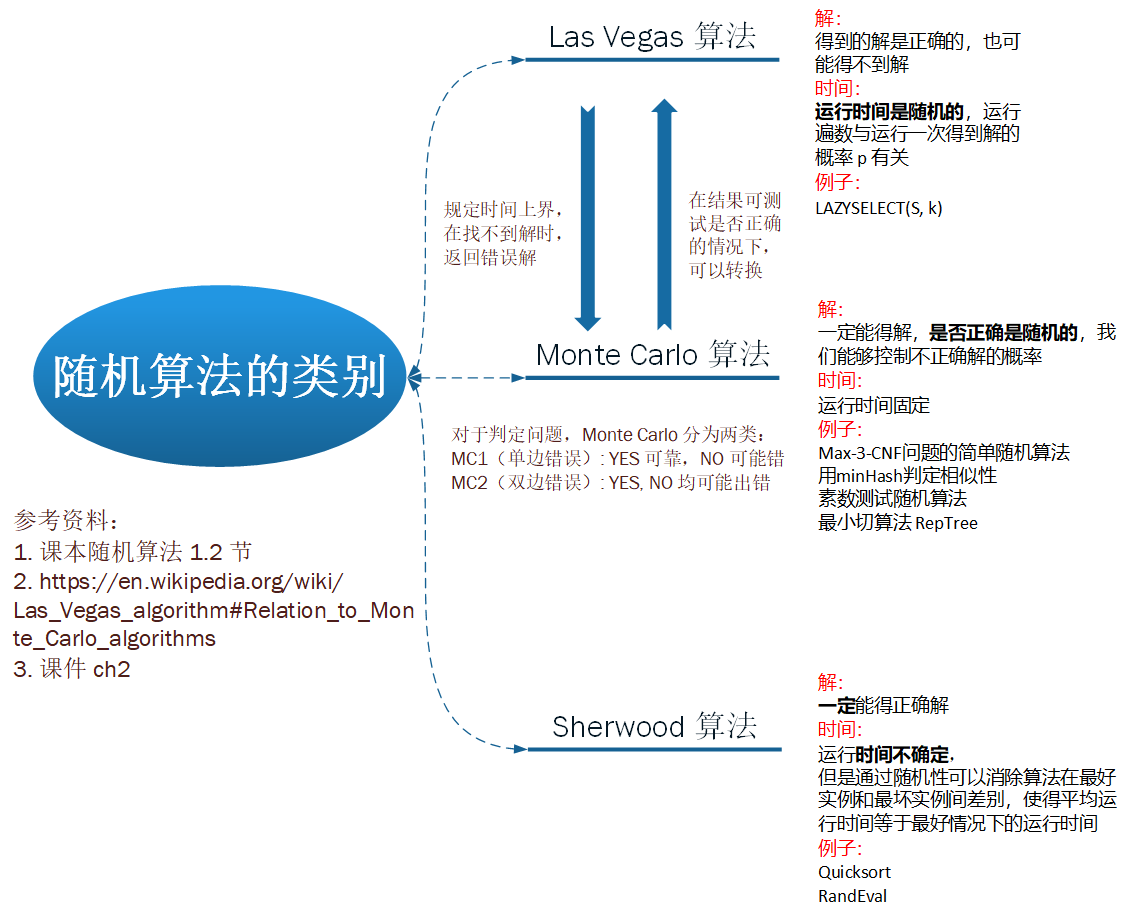# 习题一

## 1.1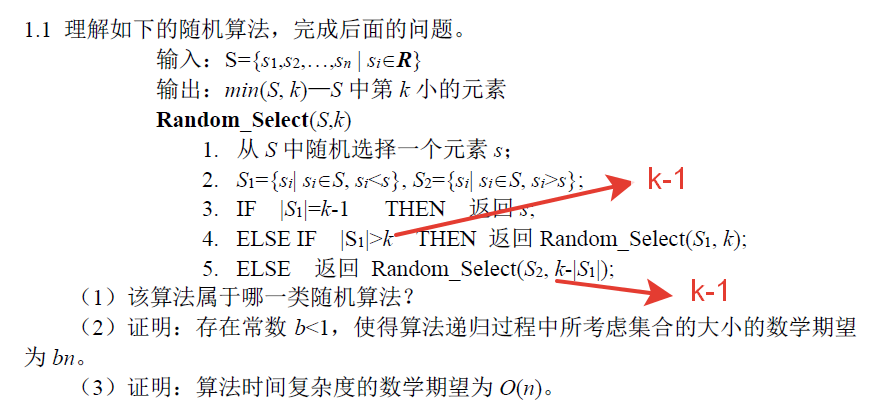(1)

(2)

1. $l < k-1$: 此时就算加上元素 $s$ 仍有 $l+1 < k$，也就是说第 $k$ 小元素一定出现在 $S_2$ 中，这对应于第 5 行。此时继续考虑 $S_2$，而 $S_2$ 的长度在 (n-k+1) ~ (n-1) 之间，平均长度为 $(n - \frac{k}{2})$
2. $l = k-1$: 此时第 $k$ 小元素一定是 $s$，这对应于第 3 行。此时算法结束。
3. $l > k-1$: 此时第 $k$ 小元素一定在 $S_1$ 中，这对应于第 4 行。此时继续考虑 $S_1$，而 $S_1$ 的长度在 k ~ (n-1) 之间，平均长度为 $\frac{k+n-1}{2}$

\begin{aligned} E(Z_2) &= \frac{k-2}{n}(n - \frac{k}{2}) + \frac{n-k+1}{n}\frac{k+n-1}{2} \\ &= \frac{2kn-k^2-4n+2k+n^2-k^2+2k-1}{2n} \\ &= \frac{n^2 + (2k-4)n - 2k^2+4k-1}{2n} \\ &< \frac{n^2 + (2n-4)n - 0+4n-1}{2n} \\ &= \frac{3n^2-1}{2n} \\ &< \frac{3n}{2} \end{aligned}

$E(Z_2) = (\frac{1}{2}+t-t^2)n + (2t-2) - \frac{1}{2n} < (\frac{1}{2}+t-t^2)n$

$b = \frac{1}{2}+t-t^2$ 即可, 此时 $b \in [\frac{1}{2}, \frac{3}{4}]$，则有 $E(Z_2) < bn$

$E(Z_i) < b^in, i \ge 2$

(3)

\begin{aligned} T(n) &= E(Z_1) + E(Z_2) + \cdots + E(Z_m) + \cdots \\ &< n + bn + b^2n + \cdots b^{\infty}n \\ &= \frac{n}{1-b} \\ &= O(n) \end{aligned}

(4)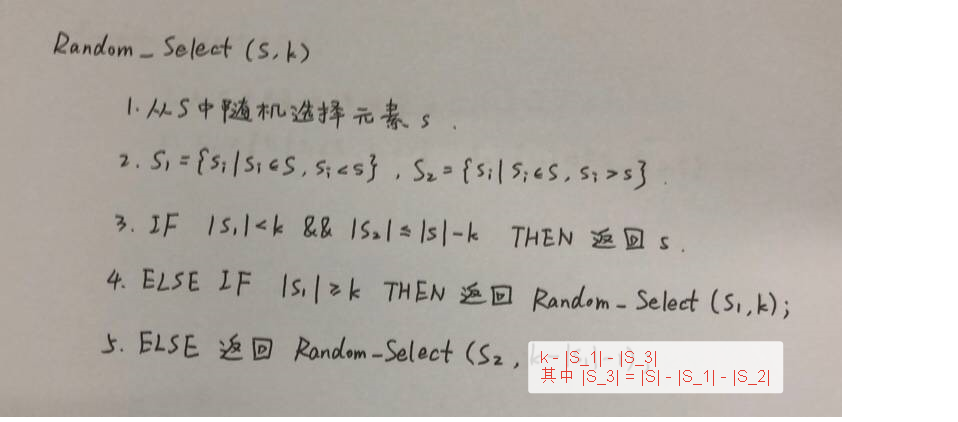## 1.2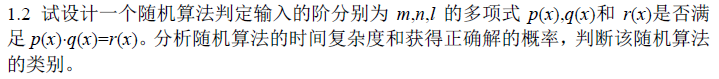$d = \max(m + n, l)$，从 $\{1, \cdots, 100d\}$ 中均匀随机地选取一个整数 $t$。计算 $s(x) = p(x)q(x) - r(x)$ 的值。如果 $s(t) = 0$，则算法判定等式成立；否则，算法判定等式不成立。

1. 若等式成立，对于任意的 $t$，都有 $s(t) = 0$，算法将给出正确的结论
2. 若等式不成立，算法发现 $s(t) \neq 0$，则给出正确的结论
3. 若不等式成立，但是算法发现 $s(t) = 0$，也就是说 $t$ 恰好为 $s(x) = 0$ 旳根，由于 $s(x)$ 的次数不大于 $d$，那么 $s(x) = 0$ 旳根的数量 $m \le d$，所以这种情况出现的概率 $P(error) = \frac{m}{100d} \le \frac{d}{100d} = \frac{1}{100}$

## 1.3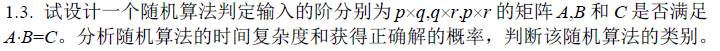$error$ 事件等价于事件 $D_{p\times r} = AB - C \neq 0$ 但是 $D\overrightarrow{s} = 0$，由于 $D \neq 0$，所以其必有某个非零元素，不失一般性，设非零元素为 $d_{11}$。考虑 D 第一行乘以 $\overrightarrow{s}$ 第 1 列（注意 $\overrightarrow{s}$ 只有一列）：

$\sum_{j=1}^nd_{1j}s_j = 0$

$s_1 = - \frac{\sum_{j=2}^nd_{1j}s_j}{d_{11}} \tag{1}$

1. $B\overrightarrow{s}: O(q\times r)$
2. $A(B\overrightarrow{s}): O(p\times q)$
3. $C\overrightarrow{s}: O(p\times r)$

## 1.4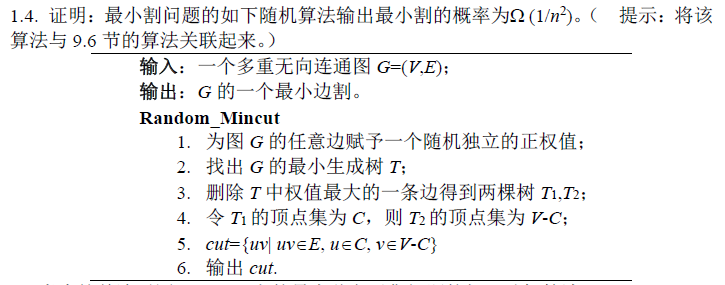1. 很显然，得到的 cut 一定是一个割。因为去掉 cut 中的边后图 $G$ 被分为了 $C$$V-C$ 两个顶点子集构成的图，两图之间再无边连接。
2. 接下来只需证明 cut 是边数最少的割。

（否则，如果某一步中最小割集的边 $u_0$ 被加到了 $T$ 中，最终输出结果 cut 必不包含 $u_0$，不是最小割集）

\begin{aligned} P[\cup_{i=1}^{n-2}A_i] &= P[A_{n-2}|\cup_{i=1}^{n-3}A_i] \\ &\cdot P[A_{n-3}|\cup_{i=1}^{n-4}A_i] \\ &\vdots \\ &\cdot P[A_2|A_1] \\ &\cdot P[A_1] \\ &\le (1 - \frac{k}{\frac{nk}{2}})(1-\frac{k}{\frac{(n-1)k}{2}}) \cdots \\ &= \frac{n-2}{n} \cdot \frac{n-3}{n-1} \cdot \frac{n-4}{n-2} \cdots \frac{1}{3} \\ &= \frac{2}{n(n-1)} \\ &\ge \frac{2}{n^2} \end{aligned}

$P[\cup_{i=1}^{n-2}A_i] \ge \frac{2}{n^2} = \Omega(\frac{1}{n^2})$

## 1.5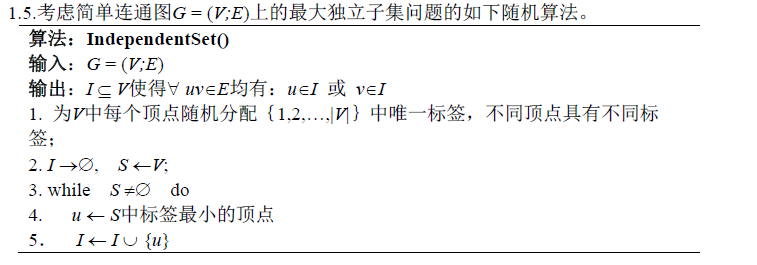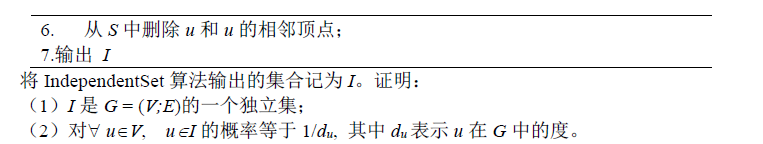(1)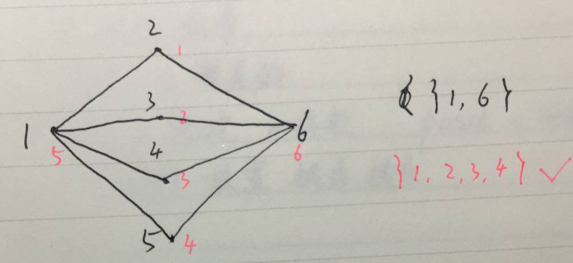(2)

$u$ 的所有邻接点分别为 $v_1, v_2, \cdots, v_{d_u}$，将 $$ 按照标签大小从小到大排序之后的序列为 $$，因为『上述顶点集中$u$ 的标签最小等价于 $u \in I$』：

$P(u \in I) = \frac{P(i_m = 0)}{P(i_m=0) + P(i_m=1) + \cdots + P(i_m=d_u)} = \frac{1}{d_u+1}$

## 1.6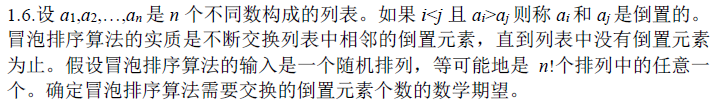1, 2 0
2, 1 1

1, 2, 3 0
1, 3, 2 1
2, 1, 3 1
2, 3, 1 1 + 1 = 2
3, 1, 2 2
3, 2, 1 2 + 1 = 3

$I_{ij} = \begin{cases} 1 \quad (a_i, a_j) 是逆序对 \\ 0 \quad (a_i, a_j) 是正序对 \end{cases}$

$E[I_{ij}] = \frac{1}{2}$

$E[\sum_{i=1}^n\sum_{j>i}^nI_{ij}] = \sum_{i=1}^{n}\sum_{j>i}^n\frac{1}{2} = \frac{n(n-1)}{2}\frac{1}{2} = \frac{n(n-1)}{4}$

## 1.7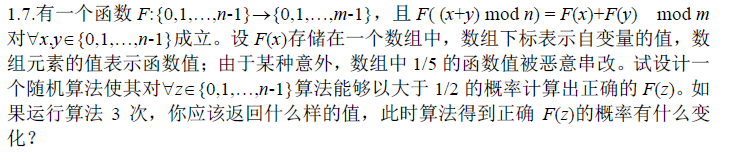$F( (x+y) \mod n) = F(x)+F(y) \mod m$

$C_3^2p^2(1-p) + C_3^3p^3 \le 3 \times (\frac{2}{5})^2\times\frac{3}{5} + (\frac{2}{5})^3 = \frac{44}{125} = 0.352$

$(C_3^2-1)p^2(1-p) + C_3^3p^3 \le 2 \times (\frac{2}{5})^2\times\frac{3}{5} + (\frac{2}{5})^3 = \frac{32}{125} = 0.256$

## 课堂练习

$\min_{A \in \mathcal{D}} A 在 I_p 上的期望运行时间 \\ \le \max_{I\in\mathcal{I}} R 在 I 上的运行时间$

（下文在输入数据量为 n 的前提下进行讨论。）

1. 排序的目的就是输出原序列一个排列，总排列数为 $n!$，其中只有一个排列是唯一正确的答案。
2. 算法通过一系列比较的答案 YES 或 NO 来推断出 $n!$ 个排列中正确的那一个，而『对手』却想方设法构造不好的输入让算法进行最多次数的比较才能得出答案。
3. 初始排列集合为 $S$，满足 $|S| = n!$，每次比较根据回答的结果是 YES 还是 NO，可以将 $S$ 划分成两个子集。
4. 『对手』总是给出划分之后较大的那个集合，这样算法最多只能将 $S$ 大小变为原来的 $\frac{1}{2}$
5. 算法结束的条件是 $|S| = 1$，因此必须至少进行 $\log_2(n!)$ 次比较。

1. 如果决策树是完全平衡的，也就是说任意两个叶子结点的深度最多相差 1，由于每个叶子结点深度都为 $\lfloor\log_2(n)!\rfloor$ 或者 $\lceil\log_2(n)!\rceil$，得证。
2. 我们只需证明，在所有叶子结点数量相同的树中，只有完全平衡树的平均深度才是最小的。对于某个不平衡的树，我们将两个最深的兄弟叶子结点移为最浅叶子结点的孩子。因为最深和最浅深度之差至少为 2，这个操作将平均深度减少了。定量来讲，最深、最浅叶子深度为 $D, d$，那么我们减少的深度是 $A = 2D + d$，增加的深度是 $B = 2(d+1) + D-1$，很显然 $A > B$。经过不断重复操作，我们可以得到一个完全平衡树，这个完全平衡树的平均深度小于原来的非平衡树的平均深度。得证。